# Overo for System Identication

228 views

### peabody124

Feb 11, 2013, 12:02:14 PM2/11/13
to
So this was kind of fun.  I modified the code to apply a pseudo-binary random sequence on the outputs.  Obviously this results in a bit of a crazy flight:

Then I fit an armax model from each output channel to each gyro axis.  I'm not entirely sure I trust my fits yet but the results are interesting.  From the pattern of signs of the kernels it's clear it's at least identifying which motor is which correctly.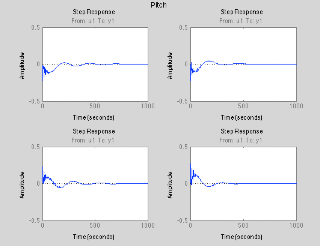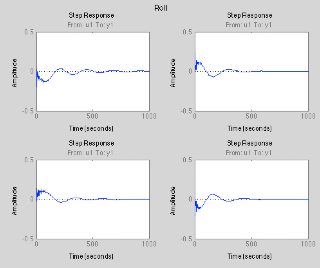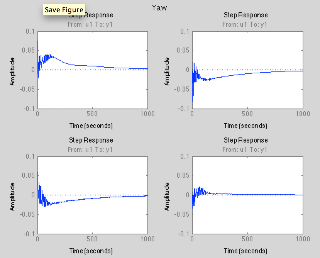Here is the data if anyone wants to analyze it: https://dl.dropbox.com/u/6645063/log_20130204_064517.mat .  There is a new object "SystemIdent" which is the superimposed signal for each channel (pre output scaling so it has an arbitrary scale).

### peabody124

Feb 16, 2013, 1:19:41 AM2/16/13
So I've got a kalman filter running that estimates the torque on the frame and roll rate (as well as attitude) for a multirotor: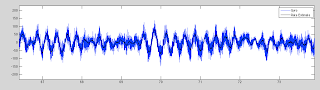You can see it really denoises the gyro trace a lot without introducing any latency.  I'm definitely tempted to code it up and try using that signal in the PID controller instead of the raw gyro.  If nothing else it will greatly reduce the noise on the output which will reduce power consumption a lot.  If that works possibly it can be used for a nicer controller than PID.

### JamesL

Feb 16, 2013, 1:57:51 AM2/16/13
i'm not sure if I understand correctly..

I have been seen our uav team doing SysID for their specific airplane to achieve best performance... afaik... the limitation of SysID is that each(different) craft has its own character.. it is not so much universal when applying it to a different config/system...  are we going to establish a sysid analysis toolset (like AQ) to characterize each specific config?

kalman filter definitely has the best performance for predicting the future state.(which i believe will increase stability a lot)....

( and i'll admit i totally forgot what i wanted to say at the beginning :(

### Kenn Sebesta

Feb 16, 2013, 6:22:48 AM2/16/13
Looks nice. I remind that the reason why the EKF considers the gyros as inputs is because it makes for a cheaper filter. In practice this works, but you've got a nice demonstration of the limitations of this. By considering angular speed as a state you get all the benefits of the filter, and the results are as stunning as expected.

Since we haven't got unlimited power, I would look at estimating bias coefficients with an LPF, thereby reducing the EKF order by as much as you would increase it with the additional states.

### peabody124

Feb 16, 2013, 12:08:58 PM2/16/13
This is actually an independent one axis filter with four states that will be integrated into the attitude controller.  Honestly right now I'm just shooting for a proof of concept.  There are two free parameters in the filter - one relating to the time constant of the ESC/prop (first order low pass) and another that scales from the output units (arbitrary -1,1) to torque normalized by moment of inertia.  Then it's just trivial

state = [angle rate prop bias]
observation = [angle(from CF) gyro]
state[t+1] = [1 dt 0 0; 0 1 dt dt; 0 0 (1-alpha) 0; 0 0 0 1] * state[t] + [0 0 alpha*gain 0]' * desired;
y[t] = [1 0 0 0; 0 1 0 0] * state[t];

I also tried using LQR to write an angle controller (back to zero) but it doesn't produce sensible outputs:
% so we have state at each step in time
% want to solve for control output that gets to
% [0 0 0] 20 steps out

Q = diag([10 10 1e-5 0]);
R = 1;
N = 20;
A = F; % use dynamics from previous step
B1 = B * g;

for j = N:-1:1
if j == N
P = Q;
else
P = Q + A'*(P - P*B1*(R + B'*P*B1)^-1*B1'*P)*A;
end
end
K = (R + B1' * P * B1)^-1*B1'*P*A;
for i = 1:size(state,1)
u_computed(i) = -K * state(i,:)';
end

### peabody124

Feb 16, 2013, 2:17:57 PM2/16/13
Oh damn, got the rate estimator running on board: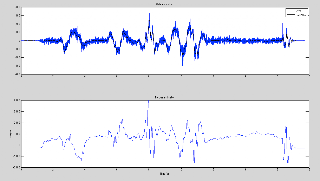and it's looking really nice.  I think I'm going to be a bit crazy and try plugging that into the PID controller and see what happens.  If you don't hear back soon it was bad and my quad attacked me!

### Y Jung

Feb 16, 2013, 2:27:41 PM2/16/13
Be care, expecting wonderful result.

### peabody124

Feb 16, 2013, 3:12:23 PM2/16/13
Ok, it flew pretty well.  I did have to increase the Kd term.  From looking at the traces it doesn't appear there is any latency but that would be my normal reason to think Kd was needed.  The good news is since the Kd is processing the smoothed trace it's a lot less noise-inducing than normal.

Here is a video of it flying: https://vimeo.com/59808533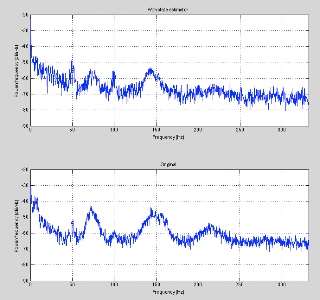Here is the spectrum of the output with the state estimator and with the raw gyro.  You can see the spectrum is lower at most frequencies (especially the peaks) with the state estimator.  I'm definitely curious to measure the power consumption at a hover in the two conditions since applying a smoother signal to the motors should be more efficient

### peabody124

Feb 16, 2013, 4:05:51 PM2/16/13
Ok, more tuning and I got the outer Kp to 10 which is pretty crazy.  It flies well though:

Really snappy responses with little overshoot.  Impressive tracking of the sticks: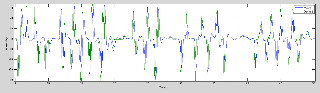And pretty little disturbance around a hover: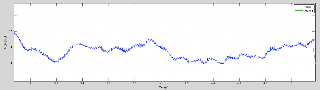We definitely might be on to something here.

### Kenn Sebesta

Feb 17, 2013, 10:54:06 AM2/17/13
A couple things:

1) Why is the bias term being multiplied by dt? Normally bias would be in deg/s, not deg/s^2.
2) The proposed system is not controllable. (See www.ece.rutgers.edu/~gajic/psfiles/chap5traCO.pdf for rigorous definition)
3) In easier terms, system is not controllable because x_4, the bias, cannot directly be affected by the control u_1.
4) The matrix Q *must* be semi-definite positive. In this case, Q(4,4) is 0, meaning that the inverse does not exist and thus not all eigenvalues are positive.

An easy solution to this is to take the bias out of the controlled state. There's nothing wrong with controlling off of a reduced state model. For instance, I use a 17-dimension state for estimating car fuel efficiency, but only 2 of those dimensions for my optimal controller.

Lastly, Matlab has two commands which might interest you: lqrd and dlqr. dlqr creates the matrix K for a linear discrete system, but we do not in fact have a discrete system, but a continuous time system controlled discretely. In this case, lqrd is better.

Looking forward to seeing your results!

### peabody124

Feb 17, 2013, 1:19:46 PM2/17/13
The bias is multiplied by dt since it's a torque bias, i.e. it captures any imbalance between the motors.  I need it for the state estimate to behave well.

So doing what you suggested and dropping the bias state from the controller ... I'm still not happy.

`%% LQR calculation g = 25000;a = 0.01;dt = 0.0015; Q = diag([100 1 1]);F = [1 dt 0; 0 1 dt; 0 0 1-a];B = [0 0 a*g]'; R = 1e1; [K,~,e] = dlqr(A,B,Q,R,zeros(size(A,1),size(B,2)))[K,~,e] = lqrd((A-eye(3))/dt,B / dt,Q,R,dt) `

Both produce similar results.  E.g. 0.0508    0.0233    0.0051.  The numbers translate to much higher values than my standard PID (e.g. I run rate Kp = 0.003 outer Kp = 3 which would be more like [0.009 0.003] for the first two elements).  However, running it over the data produces sane results.  I'm plotting the real output from the PID controller, the LQR output, and the LQR output without using the estimate of bias for state

`pseudo_state = [state(:,1) state(:,2) state(:,3)+state(:,4)];u_computed = - pseudo_state * K'; u_nt = - state(:,1:2) * K(1:2)'; ts = (t-t(1)) / 1000; plot(ts,u_computed,ts,u_nt,ts,u) legend('LQR','LQR_NoTorque','real') xlabel('Time (s)'); ylabel('Output (au)') `

Note that I have to create a pseudostate collapsing the bias estimate with the torque estimate to control estimated torque.  Not doing this results in the output drifting away from mean zero a lot (possibly it would be more optimal but scares me for now).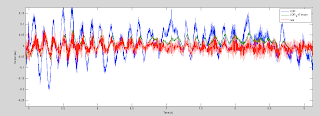It's a bit noisy to see, but the real output and the LQR coefficients without torque are pretty similar (note than depending on the values of Q and R this isn't always the case).  The real LQR output drifts around a bit still (poor capture of bias?) but more interesting is always phase advanced and higher in amplitude than the real output.  That phase advance is basically the same as adding in Kd, although now it is a nice principled (and lower noise) way to add it.

As a test of robustness I ran this on the data from the system identification, where there is a lot of perturbations added to the outputs that are not known to the controller or state estimator.  It does quite well (and looks cleaner since it's indoors)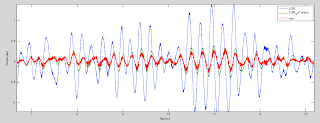So it's looking maybe doable.  I'm still not sure how confident I'll get in the rate estimator (or how easy it will be to estimate these terms for people without the overo) so we'll see if this becomes widely usable.  It would be pretty unpleasant if the rate estimator blew up or a prop came off and the torque estimator produced nonsense.

### peabody124

Feb 17, 2013, 4:29:51 PM2/17/13
Ok, I got it flying with LQR.  However, I can't find tunings that I'm particularly happy with.  It's mostly sloppy and loose control that just drifts around.  This could partly be imperfections in the state estimate.  However, I think it's much more likely that LQR just doesn't have the degrees of freedom that we're used to tuning.  For example there is no integral and you can't make it saturate the rate.  At least those are what I'm guessing.  Otherwise it seems like for just using inner and outer Kp you should be able to make it perform identically

rate_desired = outer_kp = (angle_desired - angle_current)
output = inner_kp * (rate_desired - rate_current)
= inner_kp * (outer_kp * (angle_desired - angle_current) - rate_current)
= inner_kp*outer_kp * (angle_desired - angle_current) - inner_kp * rate_current

and when I use the first two terms from my normal PIDs like that it flies - but it oscillates a lot more than in the normal mode.  That would imply the gyro smoothed estimate is slower than the real data (which is similar to what I saw using the rate estimate instead of the gyro - I needed to add some Kd).

### Kenn Sebesta

Feb 17, 2013, 4:58:22 PM2/17/13
If you look at the math, the rate loop has a P, the angular speed, an I, the absolute angle, and a D, the angular acceleration. LQR is just one fashion choosing the three constants that form the PID controller. It's an optimal fashion and so for a correctly modeled state system no better controller can be found for the given assumptions (these assumptions are encoded in the Q and R matrices). However, if you are already satisfied with the Kp, Kd, and Ki that you have found, then it would be hard to do significantly better, especially as part of a first tuning effort.

Where LQR shines is when your model is not a simple double integrator. Doubly so when the system is non-linear, as the system can always be linearized about the working point, and the optimal coefficients be found very quickly. So I would expect this to give markedly better performance on stabilizing the quad in position hold, but am not really surprised that in a first tuning effort you could not get the same performance you get out of the highly optimized PID values you've found for your quad.

### peabody124

Feb 17, 2013, 5:08:41 PM2/17/13
You're missing a degree of freedom.  I'm tuning the attitude controller which then normally has four terms: outer integral, outer kp, inner kp, and inner Kd.  LQR in my current formulation has the equivalent of the last three (ignoring rate Ki) and none of the nonlinearirities in the middle (e.g. saturating the rate desired).  However, I think the problem with LQR might be it all depends on finding the right Q and R matrices so you're still tuning - but in some weird non-intuitive place.

But it's not a total bust.  I gave up on LQR and tuned up the coefficients in the state controller manually.  Once I realize it was essentially a Kp/Kp controller I started with those coefficients.  Based on my comment earlier about the gyro latency I also reduced the noise on the gyro observation (so changed two things which maybe means LQR might work again).  I still had to add a bit of weight on the torque state to get the oscillations out.  Then I managed to get the outer Kp equivalent quite high and had a pretty good flight:

This is with the state controller on roll (sideways in most of the movie) and normal PID controller on pitch.

I would like to find Q,R matrices that produce nice results.  In a magical world those would generalize across frames so that all people have to estimate is the ESC latency and the torque/thrust ratio gain on output.

Using it for position control intrigues me too.  I was thinking about a lateral position controller.  Ignoring drag changing over velocity, the sideways acceleration at hover is  g*sin(roll).  After that the dynamics are pretty straightforward (another integrator on top of the current model).  Would you compute a lookup table of the K terms as a function of angle and then at any given instance say:

output = K(angle) * [lateral_offset; current_velocity; roll; roll_rate; torque]

### Kenn Sebesta

Feb 17, 2013, 5:11:04 PM2/17/13
Incidentally, you can throw as many integrators as you want into an LQR. It's a pretty cool feature. You simply augment the state as many times as you want with integrated state variables, and then tune the new Q and R matrices.

### Kenn Sebesta

Feb 17, 2013, 5:27:09 PM2/17/13
to
So see my previous post for how to get the additional degree of freedom. I had thought you were flying only in rate mode right now, my bad.

LQR tuning can be much more intuitive for most people. The tuning parameters have a direct link to the state and energy costs. So if you want to specifically target a single state value and minimize its error, then you simply increase that value. If you want to make the total energy in the system decrease, then increase the magnitude of R (it's mathematically identical to decrease Q's magnitude, but traditionally we increase R). If you want to get really crazy, you can even use the off-diagnonal values, which represent the coupling between the mth state variable, where m is the row index, and the nth state variable, where n is the column index.

Contrast this to cascaded LQR, where it takes a lot of experience to have a feel for what the I term on the inner loop is going to do. And cross-coupling is obviously much harder with PID, whereas with LQR it's trivial.

One thing you might do is try to figure out what Q and R will give similar parameters to the PID values you've found through experience. That would give a good starting place for tuning. Right now, you don't yet have a feel for the magnitude of the parameters with respect to each other.

As for your intuition about creating a lookup table, yes, that's exactly what you can do! Either linearize about working points offline and keep the values in a LUT, or just continually solve the ricatti equation just like is done with the EKF. Since there is enough processor power, continuous linearization would be more appropriate. Especially since the linearization of the state model is already being done with the Kalman filter so there would be no additional costs, and linearizing the input matrix is usually very quick.

### peabody124

Feb 17, 2013, 6:07:15 PM2/17/13
Ok, I found a Q and R that seems to produce reasonable results:

Q = diag([500 1 1e-2]);
R = 1e6;

Basically the cost for developing torque was too high (originally 1e-2 was 1).

I'm still not going to agree it's intuitive since I don't know how to compare a "500" penalty for degrees of error to a "1e-2" error for torque (in units normalized by inertia relative to full scale output).  But I don't think PID settings are intuitive for new people before they get used to them.  However, normally if people have sloppy control -> raise Kp.  Oscillation -> low rate Kp.  Trying that logic on the Q matrix didn't get me far.

Off to go fly these settings.

BTW I assume LQR wouldn't work as well for navigation where you say want your final state to be 100 m away still, but you don't want that large position error to make it attempt a velocity of 1000 m/s.  It seems like once you have those kind of constraints you really have to move into MPC, or is that not the case.

### peabody124

Feb 17, 2013, 6:14:45 PM2/17/13
BTW I'm still not seeing how to add integral (which would be nice since I keep having residual error).  If I create another state integrated angle - then there is nothing to constrain it.  It will just wander off and drive the output ridiculously high.  I'm guessing this is some kind of state that is accumulated error?  I just don't see the dynamics and observations for the kalman filter.

### peabody124

Feb 17, 2013, 11:25:25 PM2/17/13
to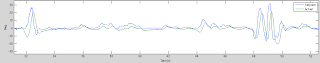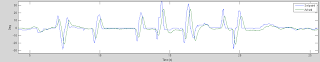So you can see it was tracking really well.  About 150 ms of latency between control and what it achieves.  You can also see the steady state error is consistently in one direction and I think this is where some integral action is required.

Any suggestions for how to try and estimate the two parameters of the KF (latency and gain)?  I remember previously making an augmented state EKF for parameter estimation but I can't find any papers/chapters on the technique.

### Kenn Sebesta

Feb 18, 2013, 4:53:36 AM2/18/13
to
What you're looking for is called state constraints. (semantics.) This is currently an apples-to-oranges problem as you're comparing cascaded control with using the full state for feedback. State constraints on full state feedback are extremely difficult to handle, as we cannot know at time t_0 what our control inputs are at time t_k that might lead to constraint violation at time t_k < t < t_inf. (Cascaded control solves this by saturating the cascaded control-- which is seen as a state by a higher level of the loop--, but with the side-effect of making the full system no longer respond like the designer intended.)

One possible strategy is to avoid forcing a mandatory absolute limit in favor of penalizing the state as it gets close to the design limits. Effectively you have a term that exponentially adds to the cost function as the state nears the bounds. This is a very effective strategy but it is no longer LQR as the cost function's PI is no longer x'Qx + u'Ru.

Another strategy is to analyze the system response and employ an iterative response based on the simulation response. This is an easier approach, as it simply involves comparing maximum actual results with maximum design limits. (Googling iterative LQR design returns http://teal.gmu.edu/~gbeale/ece_620/discrete_lqr_02/discrete_lqr_02.html, which I find to have a very nice description.)

However, no linear controller is ever going to respond well to a situation where you're asking it for a maximum final error of < 1 but the current error is >10,000 (image the units as meters to see where this kind of state "error" can arise.). Especially not if you have an integrator! This is a situation where you can either use input shaping so that the controller doesn't see the large positional error (ugh, that integrator is still going to get out of control), or you switch to a different control model.

Intuitively, we want to switch to a different control model, as when traveling long distances we are no longer concerned with our placement, but with our error from a planned time and/or path. So for hover LQR across SE(3) works great, but for trajectories through SE(3) I would choose a strategy much like we have now.

=====

For general tuning, if there are rapid oscillations in pitch then it's clear that the pitch value is being weighted too much vs. the pitch rate value. So turn down the Q value that corresponds to pitch. If you turn that value all the way down to 0*, then you effectively have a rate controller.

Since you only have one control input right now, you can simply leave R at one if it makes it easier to think about tuning.

Choosing an appropriate starting point helps with LQR. A good candidate is Bryson's method, which normalizes each state's contribution to the cost function. Do this by taking Q = diag(1/x_max_i^2) and R = diag(1/u_max_j^2), for i=1..n and j=1..m. In other words, an initial good choice is taking the diagonal elements of Q(R) equal to the inverse of the square of the state (control) maximum design value.

I find this to be much more intuitive esp. for starting out. With a PID, the starting parameters is very much a function of trial and error, or mathematical analysis. With LQR, you're designing in the time domain vs. the frequency domain, which is a much more natural link with the way we experience the world.

* Correction: Q can de semi-positive definite, but R has to be positive definite.

### Kenn Sebesta

Feb 18, 2013, 4:57:26 AM2/18/13
I'm not following why it wanders off and drives the output high. I think what you mean is that when the integrated state gets arbitrarily high that this state starts driving most of the system response? This is integrator windup, so you can use the solution as you would with PID: turn down the relative weight of the integrated state. In LQR, you do this by finding the diagonal element of Q that corresponds to the integrated state and turning it down.

I'm also not clear what " I just don't see the dynamics and observations for the kalman filter." is referring to. Perhaps you're thinking of LQG control, which is an analysis of what happens when you couple an optimal controller with an optimal observer?

### Kenn Sebesta

Feb 18, 2013, 5:07:22 AM2/18/13
To be honest, I don't think I have a good suggestion in terms of trying to estimate just latency. That's probably something that also needs to be done in the frequency domain. Maybe wavelets? They're pretty good for giving both frequency and time domain responses, but I'm not aware of any references for using wavelets for this.

Are you wanting to estimate these parameters in real-time or in offline analysis? In offline analysis you can use Kalman smoothing. That's basically where you go forward through the data, and then iterate over it going backwards in time.
Otherwise, you could try calculating innovation, both in terms of phase and gain.

If you just calculate innovation, then you wrap up latency and gain errors into one parameter. This might not be the granularity you're hoping for, but if the resulting latency is low enough then it would imply that both gain and latency are within acceptable bounds.

But I don't understand how you're going to effectively estimate either of those two without ground-truth data. Right now you're depending on integrated sensor values for ground-truth. It's not a wrong approach, but it does have its limits. I'm concerned you're getting close to those now as you're looking to optimize past the sensor noise values.

### peabody124

Feb 20, 2013, 12:23:38 PM2/20/13
Yeah, I think I might be able to compare the complex frequency response in the system identification frequency sweep to the model and estimate the gain and latency actually.  I'll have to check that math out.  It should be quite robust too.

Currently I just swept the parameters for the input model until I found one that minimized the average error.  The valus seemed pretty sane so I felt ok about it.  However, I think there are online maximum likelihood estimation methods.

Regarding the control, so I'm relatively pleased that now I have a Q,R matrix that produces the same tuning as I got manually tuning the full-state feedback.  No clue if those generalize yet :).  I simulated a one axis controller for position and used LQR to compute the feedback coefficients.  Luckily they don't even change much with angle:

`%% LQR for position% state: % 1. position % 2. velocity % 3. angle % 4. rate % 5. torque %  % hovering so T*cos(angle) = 9.81 %   T = 9.81 / cos(angle) % dPosition/dt = velocity % dVelocity/dt = -kf * Velocity + T*sin(angle) = 9.81*tan(angle) ~ 9.81 * angle * pi / 180% dAngle/dt = rate % dRate/dt = torque % dTorque/dt = a*g   g = 25000; a = 0.01; dt = 0.0015; kf = 0; Q = diag([100 10 10 10 1]); A = [1 dt 0 0 0; ...      0 (1-kf) (dt*9.81*pi/180) 0 0; ...      0 0 1 dt 0; ...      0 0 0 1 dt;      0 0 0 0 1-a]; B = [0 0 0 0 a*g]'; R = 1e5;[K,~,e] = lqrd((A-eye(6))/dt,B / dt,Q,R,dt) % or more precisely as a function of angle A = @(angle) [1 dt 0 0 0; ...      0 1 (dt*9.81*tan(angle * pi / 180)/angle) 0 0; ...      0 0 1 dt 0; ...      0 0 0 1 dt;      0 0 0 0 1-a]; B = [0 0 0 0 a*g]'; R = 1e5; angles = linspace(0.01,30,50) for i = 1:length(angles)     angle = angles(i);     [K(i,:),~,e] = lqrd((A(angle)-eye(5))/dt,B / dt,Q,R,dt) end`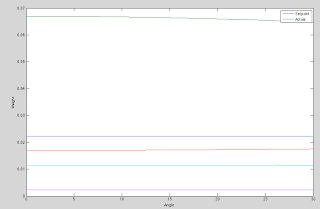However, I don't really want to implement it as is, because for now I'd like to keep the attitude controller separate from the position controller (which outputs attitude desired).  Is there a way to use the full state knowledge (and dynamics I input above) to compute the desired angle?

It sounds like what you were describing above with coupled LQR.

### Kenn Sebesta

Feb 20, 2013, 1:13:53 PM2/20/13
You want to computer the desired control angle in order to navigate your way to the set point, based on the overall error? Put another way, what I think you're asking for is a way to think of the absolute angle as the control, and not the motor speed. That's straightforward, but you'll need cascaded control on the inner loop, which basically brings us back to PID. Otherwise you're taking the derivative of the control signal, which is generally to be avoided.